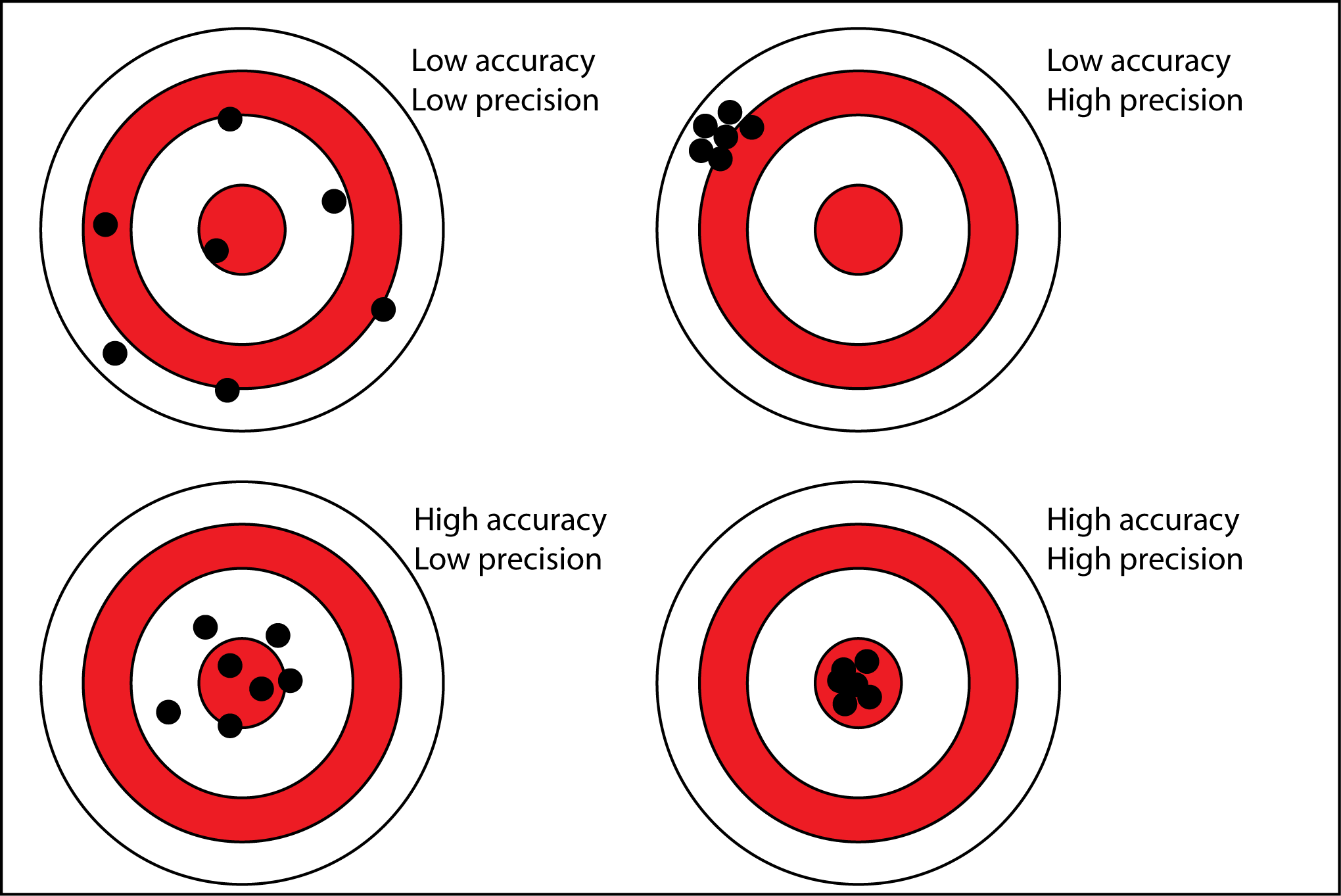# 11.1 Uncertainties and errors in measurements and results

### Qualitative & Quantitative Analysis

• Analysis of data can be classified into two types
• Qualitative Analysis
• Comes from observations and non-numerical methods
• Quantitative Analysis
• Comes from measurements and always associated with uncertainties determined by either the apparatus and human limitations such as reaction times and sight

### Uncertainties

• In science, numerical data is divided into two types
• Data with exact numbers (no uncertainty)
• Data with inexact numbers (Degree of uncertainty involved)

### Precision vs Accuracy

• Precision: Closeness of agreement between interdependent test results
• Accuracy: Closeness of agreement between the result of measurement and the true value### Significant Figures

• Number of digits reflecting precision of a given measurement
• Sometimes it is useful to write in Scientific notation
• Here are some examples:
•• Some rules for significant figures in calculation
• For multiplication or division: Result should be expressed based on measurement with smallest number of significant figures.
• For addition or subtraction: Result should be expressed based on measurement with smallest number of decimal places
• (A simple rule on the side is that your final answer should be expressed in the number of significant figures of the measurement with the lowest number of significant figures)

### Experimental Error

• Every measurement has a degree of uncertainty with it called experimental error
• There are two types of experimental error
• Systematic error: A flaw in experimental design or methodology. This affects the accuracy of the results.
• Random error: Occur because of uncontrolled variables. Cannot be eliminated. This affects the precision of the results.

### Absolute and Relative Uncertainty

• Absolute Uncertainty is the margin of uncertainty associated with the result from a measurement.
• Its symbol is given by ΔA
• Relative Uncertainty is the ratio comparing the size of the absolute uncertainty.
• Relative uncertainty = ΔA / A
• All experimental results should be reported in form:
• Experimental result = (A ± ΔA) units